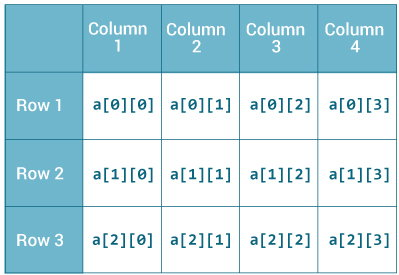# Matrix RepresentationThis type of array declaration is represented in matrix form. Memory wastage is most probable to happen in this scenario and manually unassigned blocks of memory are automatically assigned to zero.

class MatrixRepresentation {

public static void main(String[] args) {
int[][] x = new int;
x = 10;
x = 12;
x = 99;
x = 89;
x = 28;
x = 67;
x = 56;
x = 80;
x = 90;
x = 90;

for (int i = 0; i < 4; i++) {
for (int j = 0; j < 4; j++) {
System.out.println("x[" + i + "][" + j + "] = " + x[i][j]);
}
System.out.println();
}
System.out.println("The unassigned block of memory are assigned to zero.");
}
}


## Conventional Representation

This method of declaring array is often referred as conventional way of declaring array.

class ConventionalWay{
public static void main(String[] args){
int[][] x = new int; // The no of pairs of brackets represents the level of array i.e. dimensions.
}
}